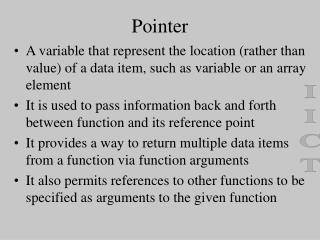DownloadDownload PresentationPointer

# Pointer

Télécharger la présentation## Pointer

- - - - - - - - - - - - - - - - - - - - - - - - - - - E N D - - - - - - - - - - - - - - - - - - - - - - - - - - -
##### Presentation Transcript

1. Pointer • A variable that represent the location (rather than value) of a data item, such as variable or an array element • It is used to pass information back and forth between function and its reference point • It provides a way to return multiple data items from a function via function arguments • It also permits references to other functions to be specified as arguments to the given function IICT

2. Pointer • It is closely associated with arrays and therefore provide an alternate way to access individual array elements. • It provides a convenient way to represent multidimensional arrays IICT

3. Fundamentals • If a data item occupies one or more contiguous memory cells, the data can be accessed if we know the location of of the first memory cell. • If v is a data item, then the address of its location can be determined by &v (here & is address operator) • We can assign this address into another variable pv=&v This new variable pv is pointer to v, since it points to the location of v IICT

4. Fundamentals Address of v Value of v • The relationship between pv and v is pv v • Data item represented by v can be accessed by expression *pv, where * is called indirection operator. (v=*pv) • If we write pv=&v and u=*pv, the value of v is indirectly assigned to u IICT

5. Example • int u=3, v, *pu, *pv; pu=&u; v=*pu; pv=&v; Address EC7 Address F8E pu u Address EC5 Address F8C pv v IICT F8E 3 F8C 3

6. Example • Int v=3,*pv,u1,u2; u1=2*(v+5) pv=&v; u2=2*(*pv+5); • Pointer variable can be assigned the value of another pointer variable (i.e pv=pu); • Pointer variable can point to another pointer variable int *p,*u,v=3; u=&v; p=&u; **p is 3; IICT

7. Example • Pointer variable can be assigned a null (zero) value, then it points nowhere pu=0 • Ordinary variables cannot be assigned arbitrary addresses &x=F8C is not permitted • Data type of the pointer must be the same as the data type of the object data. IICT

8. Passing pointer to a function • Passing arguments by reference (or by address or by location) funct(int *,int *); main() { funct(&u,&v); } funct(int *p, int *q) { *p=0; *q=0; } IICT

9. Problems • Analyzing a line of text (count no. of vowels, consonants, digits, whites paces & other characters) • Swapping two variables • Coordinate change from Cartesian to polar • Square root of a quadratic equation IICT

10. Why & is required in scanf? • char item; int partno; float cost; scanf(“%s %d %f”,item, &itemno, &cost); • Since item is the name of an array, it is understood to represent an address and does not require & • & is required for itemno and partno in order to access the addresses of these variables rather than its values IICT

11. Passing a portion of an array main () { float z; process(&z);//or process(z+50); } void process(float f[])// or void process(float *f) { } • Here 50 element of z (z to z) will be available in the process. If f is changed, then z will be affected. IICT

12. Returning pointer to the caller double *scan(double z[]); main() { double z,*pz; pz=scan(z); } double *scan(double f[]) { double *pf; pf=… return pf; } IICT

13. Pointers and one-dimensional arrays int x={10,11,12,13,14,15}; suppose the address of x is 72. here x means 12 *(x+2) means 12 &x means 76 x+2 means 76 • We can not write x++ or &x=&x • If a numerical array is defined as a pointer variable, the array element cannot be assigned initialized values IICT

14. Pointers and one-dimensional arrays • But a character-type pointer variable can be assigned an entire string as a part of the variable declaration. Thus, a string can be represented either by a one-dimensional character array: char x[]=“This is a string”; or a character pointer. char *x=“This is a string”; IICT

15. Dynamic memory allocation • We can represent an array in terms of a pointer variable and allocate memory block for the array dynamically (during the runtime of the program on the basis of user’s requirement) int *x; x=(int *) malloc (10*sizeof(int)); Or scanf(“%d”,&n); x=(int *) malloc (n*sizeof(int)); • Example: sorting a list of numbers. IICT

16. Pointers and multidimensional arrays • A multidimensional array can be represented by a lower-dimensional array of pointers int x; is same as int (*x); here x points to the first row (or first array of 20 element), x+1 points to the second 20 element array and so on. • x is same as *(*(x+2)+5) IICT

17. Pointers and multidimensional arrays • int (*x) means pointer to array of integers. Here is no. of rows is variable, but no. of column is fixed • int *x means array of pointer to integers. Here is no. of column is variable, but no. of row is fixed. Here x is equivalent to *(x+5). IICT